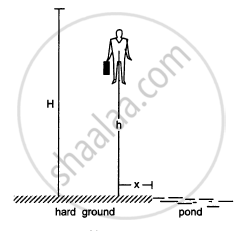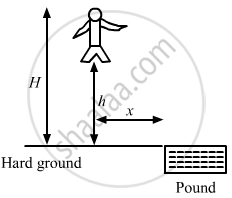Advertisement Remove all ads

# A man of mass M having a bag of mass m slips from the roof of a tall building of height H and starts falling vertically. When at a height h from the ground, the notices that the ground below - Physics

Sum

A man of mass M having a bag of mass m slips from the roof of a tall building of height H and starts falling vertically in the following figure. When at a height h from the ground, the notices that the ground below him is pretty hard, but there is a pond at a horizontal  distance x from the line of fall. In order to save himself he throws the bag horizontally (with respect to himself) in the direction opposite to the pond. Calculate the minimum horizontal velocity imparted to the bag so that the man lands in the water. If the man just succeeds to avoid the hard ground, where will the bag land?Advertisement Remove all ads

#### Solution

Mass of man = M
Initial velocity of the man = 0
Mass of bag = m

Let the man throws the bag towards left with a velocity v and himself moves towards right with a velocity V.Using the law of conservation of momentum,
$mv = MV$
$\Rightarrow v = \frac{MV}{m} . . . (1)$
$\text{ Let the total time he takes to reach ground be t_1 . }$
$\Rightarrow t_1 = \sqrt{\frac{2H}{g}}$
$\text{ Let the total time he takes to reach the height h be t_2 .}$
$\Rightarrow t_2 = \sqrt{\frac{2(H - h)}{g}}$
$\therefore \text{ The time of flying in covering the remaining height h is,}$
$t = t_1 - t_2$
$\Rightarrow t = \sqrt{\frac{2H}{g}} - \sqrt{\frac{2(H - h)}{g}}$
$= \sqrt{\frac{2}{g}}\left( \sqrt{H} - \sqrt{H - h} \right)$

During this time, the man covers a horizontal distance x and lands in the water.
$\Rightarrow x = V \times t$
$\Rightarrow V = \frac{x}{t}$
$\therefore v = \frac{M}{m}\frac{x}{t} \left[ \text{ using equation } \left( 1 \right) \right]$
$= \frac{M}{m}\frac{x}{\sqrt{\frac{2}{g}}\left( \sqrt{H} - \sqrt{H - h} \right)}$
Thus, the minimum horizontal velocity imparted to the bag, such that the man lands in the water is

$\frac{M}{m}\frac{x}{\sqrt{\frac{2}{g}}\left( \sqrt{H} - \sqrt{H - h} \right)}$
Let the bag lands at a distance x' towards left from actual line of fall.
As there is no external force in horizontal direction, the x-coordinate of the centre of mass will remain same.
$\Rightarrow 0 = \frac{M \times (x) + m \times x'}{M + m}$
$\Rightarrow x' = - \frac{M}{m}x$
Therefore, the bag will land at a distance
$\frac{M}{m}x$

Concept: Momentum Conservation and Centre of Mass Motion
Is there an error in this question or solution?
Advertisement Remove all ads

#### APPEARS IN

HC Verma Class 11, 12 Concepts of Physics 1
Chapter 9 Centre of Mass, Linear Momentum, Collision
Q 19 | Page 161
Advertisement Remove all ads
Advertisement Remove all ads
Share
Notifications

View all notifications

Forgot password?